# Moses::SquareMatrix Class Reference

A square array of floats to store future costs in the phrase-based decoder. More...

`#include <SquareMatrix.h>`

List of all members.

## Public Member Functions

SquareMatrix (size_t size)
~SquareMatrix ()
void InitTriangle (float val)
size_t GetSize () const
float GetScore (size_t startPos, size_t endPos) const
void SetScore (size_t startPos, size_t endPos, float value)
float CalcEstimatedScore (Bitmap const &) const
float CalcEstimatedScore (Bitmap const &, size_t startPos, size_t endPos) const
TO_STRING ()

## Protected Member Functions

SquareMatrix ()
SquareMatrix (const SquareMatrix &copy)

## Protected Attributes

const size_t m_size
float * m_array

## Friends

std::ostream & operator<< (std::ostream &out, const SquareMatrix &matrix)

## Detailed Description

A square array of floats to store future costs in the phrase-based decoder.

Definition at line 34 of file SquareMatrix.h.

## Constructor & Destructor Documentation

 Moses::SquareMatrix::SquareMatrix ( ) ` [protected]`

 Moses::SquareMatrix::SquareMatrix ( const SquareMatrix & copy ) ` [protected]`

 Moses::SquareMatrix::SquareMatrix ( size_t size ) ` [inline]`

Definition at line 45 of file SquareMatrix.h.

References m_array.

 Moses::SquareMatrix::~SquareMatrix ( ) ` [inline]`

Definition at line 49 of file SquareMatrix.h.

References m_array.

## Member Function Documentation

 float Moses::SquareMatrix::CalcEstimatedScore ( Bitmap const & bitmap, size_t startPos, size_t endPos ) const

Calculare future score estimate for a given coverage bitmap and an additional span that is also covered. This function is used to compute future score estimates for hypotheses that we may want build, but first want to check.

Note: this function is implemented a bit more complex than the basic one (w/o additional phrase) for speed reasons, which is probably overkill.

/param bitmap coverage bitmap /param startPos start of the span that is added to the coverage /param endPos end of the span that is added to the coverage

Definition at line 87 of file SquareMatrix.cpp.

Here is the call graph for this function: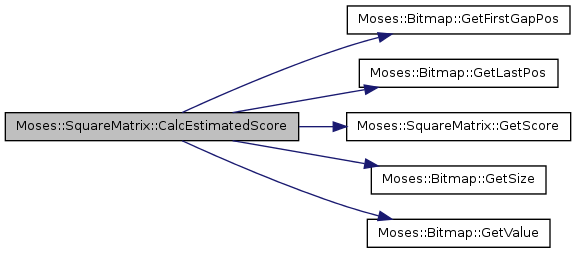float Moses::SquareMatrix::CalcEstimatedScore ( Bitmap const & bitmap ) const

Calculate future score estimate for a given coverage bitmap

/param bitmap coverage bitmap

Definition at line 48 of file SquareMatrix.cpp.

References GetScore(), Moses::Bitmap::GetSize(), and Moses::Bitmap::GetValue().

Referenced by Moses::SearchNormal::ExpandAllHypotheses(), and Moses::BackwardsEdge::Initialize().

Here is the call graph for this function: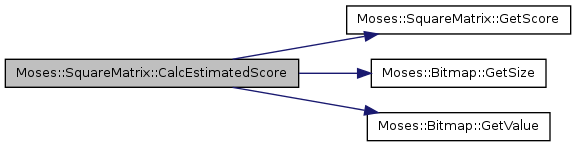Here is the caller graph for this function: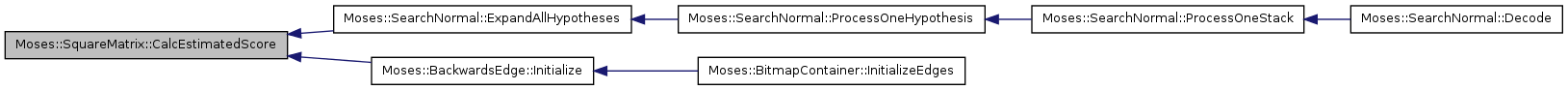float Moses::SquareMatrix::GetScore ( size_t startPos, size_t endPos ) const` [inline]`

Get a future cost score for a span

Definition at line 61 of file SquareMatrix.h.

References m_array, and m_size.

Here is the caller graph for this function: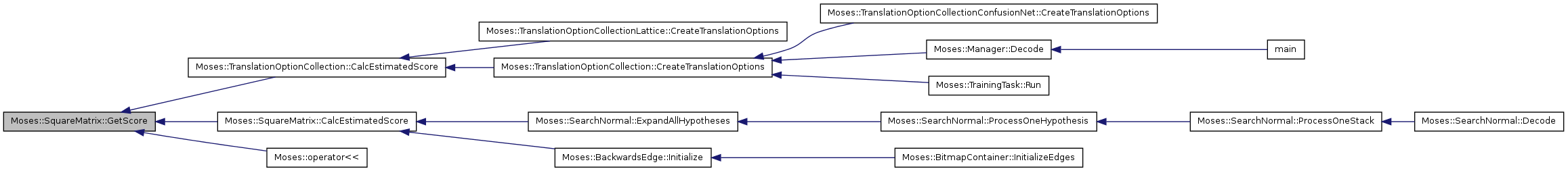size_t Moses::SquareMatrix::GetSize ( ) const` [inline]`

Returns length of the square: typically the sentence length

Definition at line 57 of file SquareMatrix.h.

References m_size.

Referenced by Moses::operator<<().

Here is the caller graph for this function: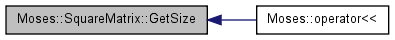void Moses::SquareMatrix::InitTriangle ( float val )

Definition at line 33 of file SquareMatrix.cpp.

References m_size, and SetScore().

Referenced by Moses::TranslationOptionCollection::CalcEstimatedScore().

Here is the call graph for this function: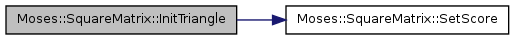Here is the caller graph for this function: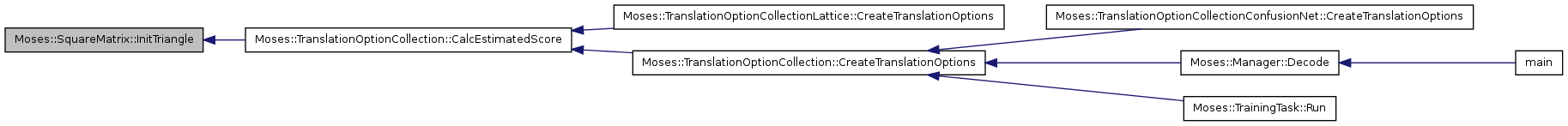void Moses::SquareMatrix::SetScore ( size_t startPos, size_t endPos, float value ) ` [inline]`

Set a future cost score for a span

Definition at line 65 of file SquareMatrix.h.

References m_array, and m_size.

Referenced by Moses::TranslationOptionCollection::CalcEstimatedScore(), and InitTriangle().

Here is the caller graph for this function: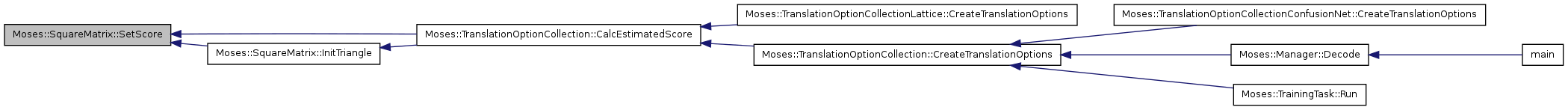Moses::SquareMatrix::TO_STRING ( )

## Friends And Related Function Documentation

 std::ostream& operator<< ( std::ostream & out, const SquareMatrix & matrix ) ` [friend]`

Definition at line 74 of file SquareMatrix.h.

## Member Data Documentation

 float* Moses::SquareMatrix::m_array` [protected]`

two-dimensional array to store floats

Definition at line 39 of file SquareMatrix.h.

Referenced by GetScore(), SetScore(), SquareMatrix(), and ~SquareMatrix().

 const size_t Moses::SquareMatrix::m_size` [protected]`

length of the square (sentence length)

Definition at line 38 of file SquareMatrix.h.

Referenced by GetScore(), GetSize(), InitTriangle(), and SetScore().

The documentation for this class was generated from the following files:

Generated on Thu Jul 6 00:33:34 2017 for Moses by1.5.9